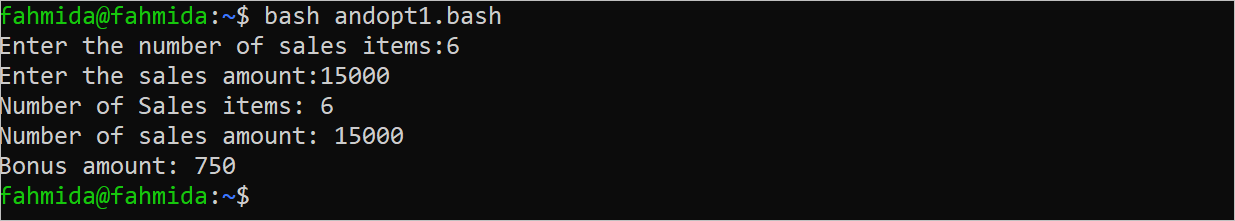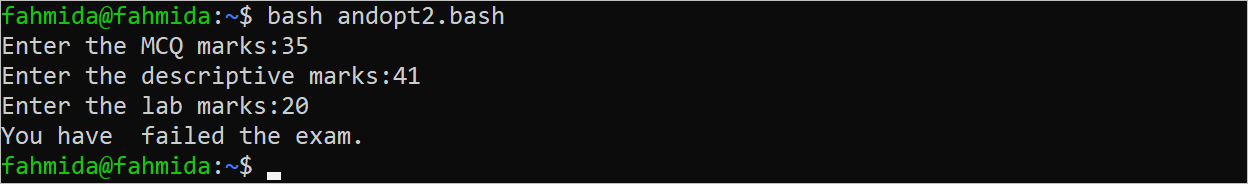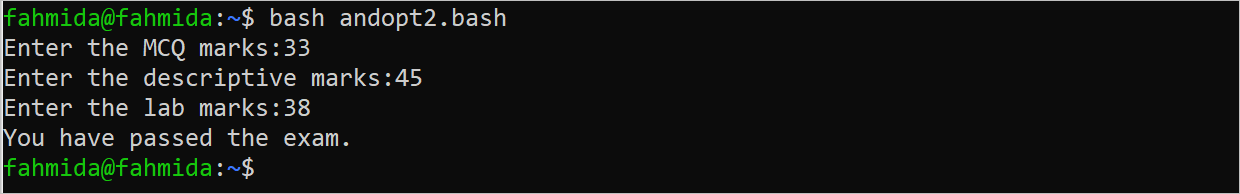| by Arround The Web | No comments

## Bash Logical AND (&&) Operator

The logical operator is used to define the logical expressions in any programming language. Commonly, three types of logical operators are used in Bash: these are AND, OR, and NOT. The AND and OR operators are used among two or more conditions. The AND operator returns true if all conditions return true. Otherwise, it returns false. The “&&” symbol is used to define the logical AND expression in the “if” condition. The uses of the AND operator in Bash are shown in this tutorial.

Syntax:

## Condition 1 AND Condition 2 AND … Condition N

The logical AND operator can be used to check two or more conditions. It returns true if all conditions return true.

## Different Uses of the AND Operator

The methods of using the logical AND operator in the Bash script are shown in this part of the tutorial.

### Example 1: Using the Logical AND Between Two Conditions

Create a Bash file with the following script that takes the total number of sales items and sales amount. Next, calculate the bonus amount based on the return value of the “if” condition. Three “if” conditions with a logical AND operator and two conditions are used in the script. If none of the “if” conditions returns true, the bonus is 0.

#!/bin/bash

#Take two inputs from the user

echo -n "Enter the number of sales items:"

echo -n "Enter the sales amount:"

#Calculate the bonus based on the output of the logical AND

if [[ \$number -ge 15 && \$amount -ge 50000 ]]; then

((bonus=\$amount*15/100 | bc -l))

elif [[ \$number -ge 10 && \$amount -ge 25000 ]]; then

((bonus=\$amount*10/100 | bc -l))

elif [[ \$number -ge 5 && \$amount -ge 10000 ]]; then

((bonus=\$amount*5/100 | bc -l))

else

((bonus=0))

fi

#Print the values of the input values with the calculated bonus

echo "Number of Sales items: \$number"

echo "Number of sales amount: \$amount"

echo "Bonus amount: \$bonus"

The following output appears if the sales items are 6 and the sales amount is 15000.The following output appears if the sales items are 25 and the sales amount is 55000.### Example 2: Using the Logical AND among Three Conditions

Create a Bash file with the following script that takes the MCQ marks, descriptive marks, and lab marks of a student. Next, three conditions are checked with the logical AND operator to print whether the student passed or failed the exam. If the MCQ mark is greater than or equal to 30, the descriptive mark is greater than or equal to 25, and the lab mark is greater than or equal to 25. Then, the “You have passed the exam” message is printed. Otherwise, the “You have failed the exam” message is printed.

#!/bin/bash

#Take three input values from the user

echo -n "Enter the MCQ marks:"

echo -n "Enter the descriptive marks:"

echo -n "Enter the lab marks:"

#Print message based on the output of the AND operator

if [[ \$m1 -ge 30 && \$m2 -ge 25 && \$m3 -ge 35 ]]; then

echo "You have passed the exam."

else

echo "You have failed the exam."

fi

The following output appears after executing the script for the values of MCQ=35, descriptive=41, and lab=20.The following output appears after executing the script for the values of MCQ=33, descriptive=45, and lab=38.### Example 3: Using the Logical AND with the Nested “If” Statements

Create a Bash file with the following script that takes the applicant ID, department name, and the post from the user. Next, the post and department values are checked with the logical AND operator. If this “if” statement returns true, the next “if” statement is checked. The output message is printed based on the returned values of nested “if” statements.

#!/bin/bash

#Take input values from the user

echo -n "Enter the applicant ID:"

echo -n "Enter the department name:"

echo -n "Enter the post name:"

#Use of AND operator in nested if

if [[ \$post == "Manager" && \$dept == "HR" ]]; then

if [ \$id == "5001" ]; then

echo "You are selected in the \$dept department."

else

echo "You are not selected."

fi

elif [[ \$post == "Accountant" && \$dept == "Sales" ]]; then

if [ \$id == "5620" ]; then

echo "You are selected in the \$dept department."

else

echo "You are not selected."

fi

else

echo "Department or Post is invalid."

fi

The following output appears after executing the script with the values of ID=”5002”, department=”HR”, and post=”Manager”.The following output appears after executing the script with the values of ID=”5620”, department=”Sales”, and post=”Accountant”.## Conclusion

The simple uses of the logical AND operator in Bash script are shown in this tutorial with multiple examples to help the new Bash programmers.

Source: linuxhint.com